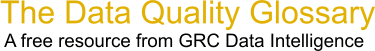Choose Index below for a list of all words and phrases defined in this glossary.

# Central Time Theorem

index | Index

## Central Time Theorem - definition(s)

Central Time Theorem - The central limit theorem states that given a distribution with a mean m and variance s2, the sampling distribution of the mean appraches a normal distribution with a mean and variance/N as N, the sample size, increases.

The central limit theorem explains why many distributions tend to be close to the normal distribution.

Here's a great learning example website: www.math.csusb.edu/faculty/stanton/m262/centrallimittheorem/clt.htmlIf you are are averaging your measurements of a particular observable, your average's distribution may seem to tend toward a normal distribution. If the random variable that you are measuring is decomposable into a combination of several random variables your measurements may also seem to be normally distributed

YOU CAN STOP HERE IF YOU DO NOT WANT THE CALCULATIONS. However, I suggest just reading the words to keep yourself safe - the stuff between the dollar signs should suffice. I hope that my notation is clear for those venturing into the formulas.

Just to be on the safe side and preclude easy misinterpretations, here are some perspectives with three Central Limit Theorems. NO PROOFS! Immediately below you have one strong theorem and one weak one. At the very bottom is a theorem that is only referenced for completion and is for those who have fun proving limits of weighted sums of L2 integrals. Except for the third theorem, I trust that this will provide everyone with more light than heat!

One Strong Central Limit Theorem states the following: The average of the sum of a large number of independent, identically distributed random variables with finite means and variances converges "in distribution" to a normal random variable. {Example: "independent" production runs for the manufacturing of a computer (or appliance) circuit component, or board; milling shafts, polishing 1000s of microscope or phased array telescope lenses (Hawaii, where are you?), software modules, etc.} One must be careful about the type of convergence, such as "convergence in measure (or almost everywhere)" vs. "mean-square convergence" vs. "convergence in distribution". {Please note: "convergence in distribution" is a much weaker than "convergence in measure", but it is also weaker than "mean-square convergence"}

So, here we go: the average of the sum of a large number of independent, identically distributed random variables X1, X2, ....., Xn with finite means M(j) and finite variances Var(j) converges IN DISTRIBUTION to a normally distributed random variable X' with a finite mean M and a finite variance Var.\$\$\$\$\$\$ The formula follows (my apologies for my notation):

X1 + X2 + X3 + ....---> X' , where X' ~ N(M, Var), i.e., Normally Distributed with finite mean = M, and finite variance Var.

" ---> " denotes "converges toward"

If for each of the Xj, M(j) = 0 and Var(j) = 1, then X' ~ N(0,1)

A Weaker Central Limit Theorem: A sequence of jointly distributed random variables X1, X2, X3, ...., Xn with finite means and variances obeys the classical central limit theorem, IF the sequence Z1, Z2, Z3, ....., Zn converges IN DISTRIBUTION to a random variable Z ~ N(0,1) (WO! BACK UP! BE VERY CAREFUL HERE! THAT WAS AN IF!! THE TABLES HAVE BEEN TURNED!!),

where

Zn = [Sn - E(Sn)]/[Std Dev(Sn)], and Sn = X1 + X2 + X3 + .... + Xn, Std Dev (Sn) = Square Root {Var(Sn)} is the standard deviation, and E(Sn) is the Expectation of Sn, the sum of the random variables Xj, 1<= j <= n.

" <= " denotes " less than or equal to"

The random variables Z1, Z2, ...., Zn are called the sequence of normalized consecutive sums of the sequence X1, X2, ...., Xn.

-----

In terms of the characteristic functions (see Section **** below), the sequence {Xj} obeys the central limit theorem, IF for every real number a:

In the limit as n goes positively to infinity, the Characteristic Function (CF) of Zn(a) converges to exp(-a^2/2)

The limit CF(Zn(a)) --> exp(-a^2/2), as n ----> infinity, where a^2 = "a squared", and exp( ) is the exponential function.

" ^ " denotes exponentiation

The gold nugget here is that the function exp(-a^2/2) is the Characteristic Function (CF) for a random variable that is distributed normally N(0,1)!

------

[Characteristic Functions, i.e., the Fourier Transforms of the probability density functions of random variables (when they exist!). However, the spectral densities (the transforms of the Distribution Functions) always exist!)]

Two important concerns: the types of convergence, and what they mean. Two random variables with exactly the same distributions will often differ from one another to the vexation of the observer. However, they will tend to hop, skip, and jump around there central moments (i.e., means, variances, etc.) similarly.

------

Two important cases (Recommendation: Leave Case 2 for those who are most comfortable with probabilistic L2 calculus):

Case 1. Independent, identically distributed random variables X, and {Xj} with finite means M, and M(j) and variances Var, and Var(j).

Then for Zj = [(X1+....+Xj) - jE(X)]/[Sqrt(j)Var(X)], j=1,.....n,..... The limit of the characteristic function for Zj will converge to a normal characteristic function.

" * " denotes multiplication

Case 2. Independent random variables with finite means and (2 + delta)th central moment {i.e. a little bit more exponentiation than the variance's square}. Delta is some very small number, and the (2 + delta)th central moment for Xj = mu(2+delta; j) = E[|Xj - E(Xj)|^(2 + delta)]. Please recall E[g] is the expectation of g.

If the {Xj} are independent and the Zj are defined as in Case 1, the characteristic functions (CFs) will converge to the normal CF exp(-a^2/2), IF the Lyapunov Condition holds:

The Lyapunov Condition:

In the limit as j goes to infinity {1/Var(2+delta)[Sj]}{Sum(mu(2+delta; j)|1<=j<=n)} = 0, where

Var(2+delta)[Sj] = E[|Sj - E(Sj)|^(2 + delta)]

[Category=Data Quality ]

Source: iSixSigma, 05 January 2011 09:13:28, https:web.archive.org/web/20111109014246/http:www.isixsigma.com/index.php?option=com_glossaryData Quality Glossary.  A free resource from GRC Data Intelligence. For comments, questions or feedback: dqglossary@grcdi.nl